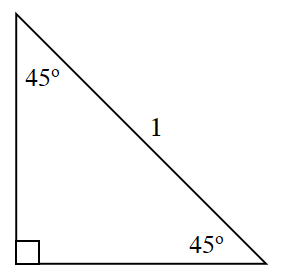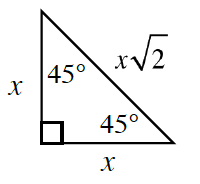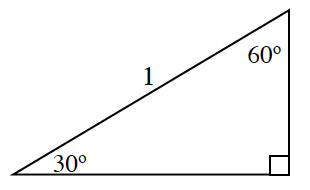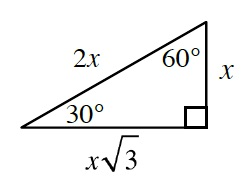### Home > PC3 > Chapter 2 > Lesson 2.1.3 > Problem2-38

2-38.

​​Copy the triangles below and determine the exact values of the missing sides. Each triangle has a hypotenuse of one unit.

1.$x\sqrt{2}=1$

1.$2x=1$